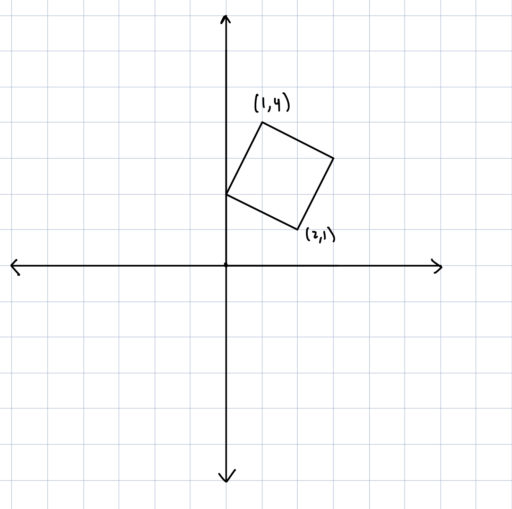Geometry questions sometimes involve various graphs. For example:

Example 1
Find the area of the following square: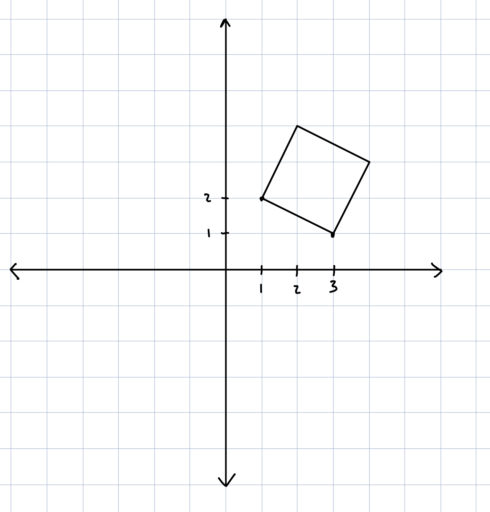Now, these problems do not differ substantially from what we have before. Essentially, graphing problems give you the same information in a slightly different way. So instead of telling you how long the sides of a particular shape are, they might give you the coordinates of the endpoints. But of course, we know how to infer the length of that side, for we know the Pythagorean Theorem:

Example 2
A rectangle has a side whose vertices are at (1, 3) and (2, 2). Find the length of that side.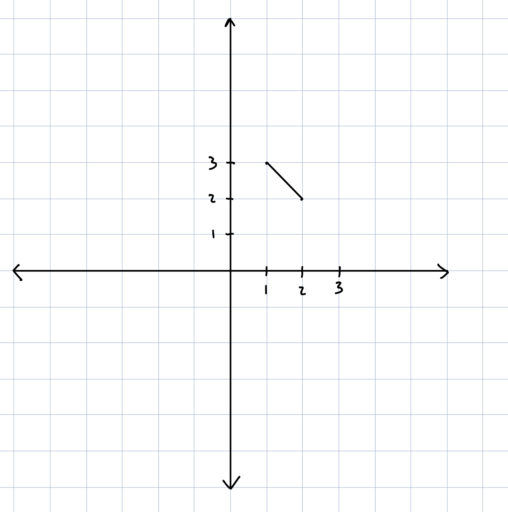In this vein, a more realistic problem might look like:

Example 3
A triangle has vertices at (1, 4), (2,3), and (0,0). Find its perimeter.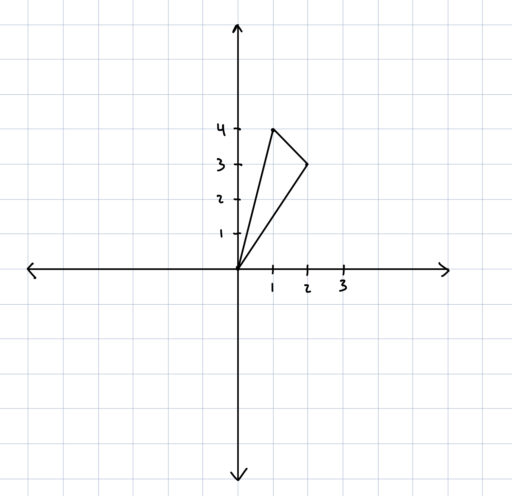Similarly, graphing problems can involve angles:

Example 4
A triangle has vertices atand. Find the degree of angle.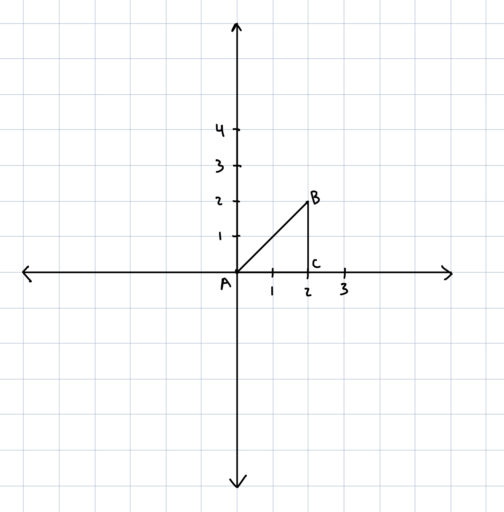In short, if you have a geometry problem that involves certain points or a diagram, you can generally use those points or that diagram to infer aspects like the lengths of certain sides or the degrees of various angles, and then use that information as you normally would.

Practice Problems:

1. A rectangle (shown below) has vertices at (0,1), (2, 0), and (2,5). Where is its fourth vertex?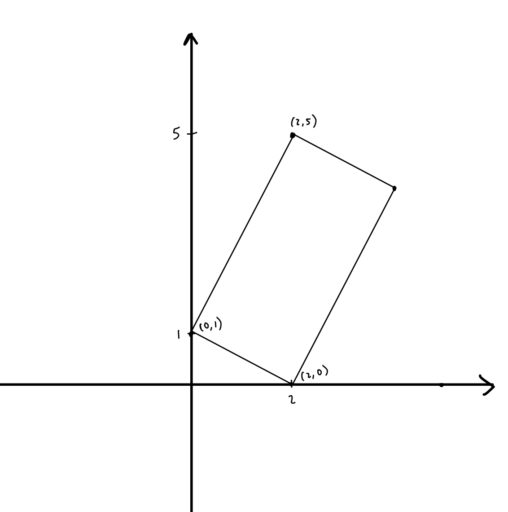2. A circle has its center at pointand its circumference includes the point. Find its radius.

3. A triangle has vertices at (1, 4), (2,3), and (0,0). Find its area.4. The square in the following diagram has points at (2, 1) and (1, 4) as labelled. What is the area of the square?